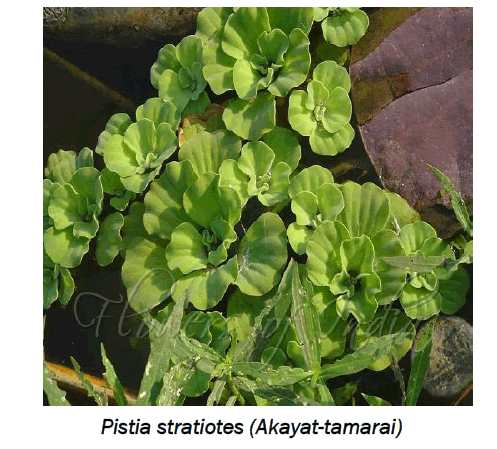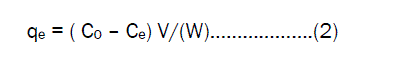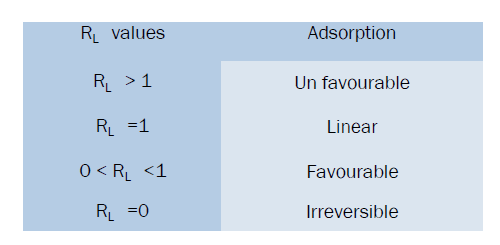e-ISSN: 2319-9849

All submissions of the EM system will be redirected to Online Manuscript Submission System. Authors are requested to submit articles directly to Online Manuscript Submission System of respective journal.

# Equilibrium and Thermodynamics Studies on the Removal of Iron (III) Onto Activated Pistia Stratiotes Leaves Nano Carbon

S Arivoli*, V Marimuthu and T Rose Judith

Department of Chemistry, Thiru. Vi. Ka. Government Arts College, Thiruvarur, Tamil Nadu, India

*Corresponding Author:
S Arivoli
Department of Chemistry, Thiru. Vi. Ka.
Government Arts College, Thiruvarur, Tamil Nadu, India

Received: 28/09/2013; Revised: 19/11/2013; Accepted: 21/11/2013

Visit for more related articles at Research & Reviews: Journal of Chemistry

## Abstract

The present study is on adsorption of Fe (III) ions by Activated Pistia Stratiotes Leaves Nano Carbon. It uses batch adsorption techniques. The influence of contact time, initial concentration, dosage of adsorbent and effect of solution pH were investigated. The isotherm studies of RL values showed that the adsorption process was favorable. Thermodynamic parameters such as ΔH0, ΔS0 and ΔG0 were evaluated. The data indicate that, the adsorption was spontaneous and is an endothermic nature. Adsorption kinetics was tested with pseudo- second –order, Elovich model and intra – particle diffusion models. Kinetic studies indicate an adsorption pseudo – second –order reaction. This study shows that intra – particles played a major role in the adsorption of Fe (III) ions mechanism. The Activated Pistia Stratiotes Leaves Nano Carbon has high adsorption capacity and adsorption rate for the removal of Fe (III) ions from aqueous solution.

#### Keywords

Adsorption, Iron (III) ions, kinetics, Activated Pistia Stratiotes Leaves Nano Carbon, Thermodynamics

#### Introduction

The removal of heavy metals such as Pb, Fe, Cr, Cd, Co, etc., from ground and industrial water is a matter of great interest especially in countries in the limited water resources. Iron ions have attracted the greatest attention of researchers as one of the heavy metals and they are found in many manufacturing industries such as the metal finishing and galvanized pipe . The presence of iron ions in ground and industrial water becomes toxic at high level and then may cause environmental and human health problems [2,3]. The major constituents of the lithosphere is said to be iron which comprises approximately 5% of it. In most of the cities the municipal waste effluent contains iron because they manufactured iron and steel through industries. In most of part the surface level of water is said to be in the form of sediments which is formed by iron complexes with the sulphate. The primary concern about iron in drinking water reduces the taste of the water. The taste of drinking water can be easily detected even at low concentration of iron 1.8mg/L  The iron toxicity arouse many problem which is said to be include anorexia, oligura, diarrhea, hypothermia, diphasic shock, metabolic acidosis and even death. And also it causes vascular congestion of the gastrointestinal tract, liver, kidneys, heart brain, adrenals and thymus. With acute iron poisoning, much of the damage happen to the gastrointestinal tract and liver which may result from the high level of iron concentration and free radical production leading to heptatoxicity via lipid per oxidation and destruction of the hepatic mitochondria. As a result of iron storage disease, the liver becomes cirrhotic. Hepatoma, a primary cancer of the liver, has become the most common cause for death among patients with hemochromatosis  Organic pollutants, can be bio-degraded, heavy metals don’t degrade into harmless end product .

In the present investigation the adsorption of Iron ion on activated nano carbon prepared from Strychnos nux-vomica L. by carbonization with sulphuric acid has been achieved. The kinetic and equilibrium adsorption data obtained were utilized to characterize the sample prepared . The amounts and rates of adsorption

of iron using above activated nano carbon from water were then measured. Three simplified kinetic models including pseudo first order, Pseudo second order equations and Elovich equations were used to describe the adsorption process.

#### Materials and Methods

Absorbent

The Pistia Stratiotes Leaves (Akayat-tamarai) collected from nearby Thiruvarur district was activated around 1200°C in a muffle furnace for 5 hrs the it was taken out, ground well to fine powder and stored in a vacuum desiccators.The effect of various parameters on the removal of Iron (III) on to Activated Pistia Stratiotes Leaves Nano Carbon was studied. All Chemicals used were in high level purity of the commercially available AR grade. A stock solution of the adsorbate containing 1000 mg/L of Fe (III) was prepared by dissolving the 7.0200g of Ammonium Iron (III) Sulphate Decahydrate in de-ionized water. The stock solution was diluted to the required initial concentration (range 25 to 125 mg/L). In each adsorption experiment, 50 ml of metal ion solution with a known concentration was added 25 mg of Activated Pistia Stratiotes Leaves Nano Carbon in a 250 mL stopper glass flask at 30, 40, 50 and 60 °C and the mixture was stirred on a mechanical shaker of 120 minutes.

The samples were withdrawn at the appropriate time intervals and the adsorbent was separated by centrifugation at 1500 rpm for 10 minutes. The supernatant was analyzed for the residual Fe (III) concentration and was measured before and after treatment with an atomic absorption spectrophotometer (Perkin Elmer 2380).The effect of pH on the rate of adsorption was investigated using Fe (III) concentration of 50 mg/L for constant Activated Pistia Stratiotes Leaves Nano Carbon. The pH values were adjusted with 1N HCl and in 1N NaOH solution. The adsorption of process was carried out at different temperature (30, 40, 50, and 60 °C). This was helped to estimate the impact of changes of thermodynamic parameters caused by the temperature effect. The amount of adsorption at time t, qt (mg/g), can be determined using the following formula;where Ct is the metal ions liquid phase concentration(mg/L), at any time, Co is the initial concentration of metal ions in solution (mg/L), V is the volume of solution (L) W is the mass of adsorbent(g) The amount of adsorption at equilibrium qe(mg/g) was computed by using the following equationwhere C0 and Ce are the liquid phase concentration of Fe(III) ions (mg/L) initially and at equilibrium. The removal percentage of Fe (III) ions can be calculated as:where C0 is the initial concentration of the Fe(III) ions in solution (mg/L ), and Ce is the equilibrium concentration of Fe (III) ions in solution(mg/L) ,

#### Results and Discussion

Effect of contact time on Fe (III) ions adsorption

In batch adsorption process contact time is one of the potent factors. In the resultant process all of the parameters except contact time, Temperature (30, 40, 50 and 60°C), adsorbent dose (25 mg to 50 ml) and agitation speed (120 rpm), were kept constant. The maximum removal of Iron (III) by Activated Pistia Stratiotes Leaves Nano Carbon required contact time of 60 minutes, this is represented (Figure 1), It was revealed that the removal of Iron (III) increase with increase in contact time, and the optimal removal efficiency was reached within 40 minutes. There is the maximum adsorption occurs at 40 minutes after which the adsorption phase reaches an equilibrium. At this point maximum amount of iron (III) adsorbed under the particular conditions. (Figure 1), indicates that, the time variation curve is single, smooth and continuous and it shows the formation of monolayer coverage on the outer interface of the adsorbent [7-9]

Effect of Initial Iron (III) Concentration

To study the effect of initial Fe (III) ions on the adsorption level varying Concentration (25, 50, 75,100 and 125 mg/L) under the constant Temperature (30, 40, 50 and 60 0C), pH 6.5 agitation speed (120 rpm), and 25 gm of adsorbent dose (25mg/50 ml). The Removal efficiency by the effect of initial Fe(III) ions concentration was obtained from the experimental results were presented in (Table 1), It shows that the increasing initial Fe(III) concentration results decrease of the removal efficiency of Fe (III) ion. In case of low Fe (III) ion concentrations the ratio of the initial number of moles of metal ions to available surface area of adsorbent is large and subsequently the fractional adsorption becomes independent of initial concentration  .However at higher concentrations of metal ions the available sites of adsorption becomes fewer and hence the percentage removal of metal ions which depends upon initial concentration

Table 1: Equilibrium Parameters for the Adsorption of Fe (III) on APSNC Adsorbent

The effect of varying adsorbent doses was investigated by employing (25, 50, 75, 100 and 125 mg). They were agitated with 50ml of Fe (III) ions solution. The data are tabulated in Table 2. The adsorbent dosages have impact on the adsorption of Fe (III) ions by APSNC are represented in (Figure 2), It is revealed that the increased adsorbent doses with increase the Fe (III) ions removal efficiency. This is because the contact surface areas of adsorbent have increased. It would be more probable for Fe (III) ions on to be adsorbed on adsorption sites and the adsorption efficiency increased. Hence the entire studies are carried out with the adsorbent dosage of 25 mg 50 ml of adsorbate solution.

Table 2: Langmuir and Freundlich Isotherm Parameters for the Adsorption of Fe (III) on APSNC

Effect of solution pH

The Langmuir isotherm model  studied saturated monolayer coverage of solute on the surface of the adsorbent. The linear expression of Langmuir isotherm model was applied in the formCe is equilibrium constant of metal ions (mg / L), qe is the amount of metal ions adsorbed at equilibrium (mg/g), Qm is the constant related to maximum adsorption capacity (mg/g), b is the Langmuir constant related to energy of adsorption. From the equation the linear plot of Ce /qe against Ce should be a straight line. It shows that adsorption obeys the Langmuir isotherm model. The constant Qm and b can be calculated from slope and intercept of the plot and the values are given in Table.2.The shape of the Langmuir isotherm can be expressed by dimensionless factor, RL [13,14] .The RL values give an idea about the nature of the adsorption process as given below.The RL values were 0 to 1for the present investigation. RL values given in table.3. The results indicate Langmuir isotherms model fits very well for the selected adsorbate and adsorbent system.

Table 3: Dimensionless Separation Factor (RL) For the Adsorption Of Fe (III) on APSNC

Freundlich Isotherm

The metal ions distribution between the liquid phase and solid phase can be described by using the Freundlich isotherm model  .The well-known equation for the Freundlich isotherm model is given asWhere qe is the amount of Fe(III) ions adsorbed in per unit weight of sorbent (mg/g) , kf is the Freundlich constant, which is related to measure of adsorption capacity(mg/g).1/n is the sorption intensity(mg/L) and Ce is the equilibrium concentration(mg/L). Linear plots of log qe against log Ce The Kf and 1 / n values can be obtained from the slope and intercept respectively and the values are tabulated in table.2. When 1/n lies between 1 to 10 values the linearity of Freundlich plot suggests the formation of multilayer of Fe(III) on the adsorbent surface. The Freundlich adsorption coefficients Kf of Fe (III) on Activated Pistia Stratiotes Leaves Nano Carbon was found to be 4.7951, 4.8733, 4.4842 and 5.0665 mg /L. from the values of 1/n and Kf confirm plaster of pairs is more efficient in the removal of Fe (III) ions.

Thermodynamic parameters

Thermodynamic parameters such as standard free energy (ΔG0) standard enthalpy change (ΔH0) and standard entropy changes (ΔS0) were calculated by using equilibrium constant (K0) change with temperature (T).The free energy change can be determined by the following equation .Where ΔG0 is the free energy change of sorption process (kJ/ mol), Ko is the equilibrium constant, T is the temperature in (K), R is the universal gas constant. The free energy change may be expressed in terms of enthalpy change of sorption as a function of temperature as followsThe adsorption coefficient K0 can be obtained by combined and rearranging Eqs (6) and (7)where ΔH0 is the standard heat changes of the sorption,ΔS0 is the entropy change of sorption(KJ/mol). The standard enthalpy and entropy changes values are evaluated from the slope and intercept of linear plot lnk0 against 1/T. Thermodynamic parameter values are obtained from the equation (8) for the sorption of Fe (III) ions on Activated Pistia Stratiotes Leaves Nano Carbon and the values are tabulated in Table.4. The negative values of free energy changes confirm the spontaneous nature of sorption of Fe(III) on Activated Pistia Stratiotes Leaves Nano Carbon and the positive values of ΔH confirm the sorption process of an endothermic nature. The positive values show increased randomness at solid –solution interface during the sorption of metal ions on the Activated Pistia Stratiotes Leaves Nano Carbon.

The Pseudo second-order model

The pseudo – second – order rate expression based on the sorption capacity of solid phase is generally expressed  aswhere k2 is the rate constant of second order adsorption (g.mg-1.min-1), For the same boundary condition the integrated form of eqs (9) becomesIf the initial sorption rate h (mg.g-1 min-1) at t= 0 is defined aswhere the k2 and h values can be calculated from the slope and intercept of the plots of (t/qt) versus t The pseudo- second- order rate constant k2 values, h value and correlation coefficient (γ) values are presented in table. 5. The fair degree of agreement between the calculated values and experimental values were found in the pseudo- second – order model. The correlation coefficient for the adsorption of Fe(III) was found very high(γ = 0.99) .This value indicates that, the adsorption capacities (qe) is very close to the calculated adsorption capacity, the sorption of Fe(III) ions on Activated Pistia Stratiotes Leaves Nano Carbon follows the pseudo –second- order model.

Elovich equation model

The Elovich model [18,19] related varying adsorption surface areas and rate of adsorption. It is generally expressed as ;where α is the initial adsorption rate (mg.g-1 min-1 ),β is the desorption constant (g. mg-1), during any one experiment. Integrating this equation for the boundary condition, eqs (12) becomesIf the Fe(III) adsorption fits the Elovich model a plot of qt against ln(t) should yield a linear relationship with a slope of (1/β) and an intercept of (1/ β) ln (α β) .The Elovich equation parameters such us α , β and correlation coefficient(γ) are present in the Table.5. From the Elovich model indicates that, the initial adsorption increases with increasing temperature in all sizes of adsorbent area.

The intra-particle diffusion model

The Weber and Morris  suggest the following kinetic model to identifying the mechanism involved in the adsorption process is of an intra- particle diffusion model or not. It is generally expressed aswhere Kid is the intra-particle rate constant (mg/g/min1/2), c is the intercept, qt is the amount of metal ions adsorbed (mg/g), at time t. From the plot of qt Vs t1/2, the intra-particle rate constant can be determined from the slope of the plot. The values of C give on idea about the boundary layer thickness. The calculated intra- particle diffusion parameters present in Table .5. The γ values indicate that, intra- particle diffusion process is the controls the sorption rate.

#### Conclusion

The adsorption of Fe(III) on to Activated Pistia Stratiotes Leaves Nano Carbon was investigated in this research work. The adsorption equilibrium well correlated with both Langmuir model and Freundlich isotherm models. The adsorption kinetic process was found pseudo –second – order model. Isotherm and kinetic study indicates that the APSNC can be effectively employed for the adsorption of Fe (III) ions. Thermodynamic results show that adsorption of Fe (III) ions on to APSNC spontaneous and physical adsorption.

#### Acknowledgement

Authors are sincerely thanks the University Grants Commission, New Delhi for providing the funds from Major Research Project